• ## 前向后项差分和显式隐式欧拉法

万次阅读 多人点赞 2016-11-15 16:58:24
> 本文主要介绍前向后向差分显式隐式欧拉法及其稳定性分析。 > 前向差分对应显式方法，后向差分对应隐式方法。显式欧拉法是比较流行的显式方法，隐式欧拉法是比较流行的隐式方法。 > 显式欧拉法条件稳定，对积分...

摘要：
本文主要介绍前向后向差分，显式隐式欧拉法及其稳定性分析。
前向差分对应显式方法，后向差分对应隐式方法。显式欧拉法是比较流行的显式方法，隐式欧拉法是比较流行的隐式方法。
显式欧拉法条件稳定，对积分步长有要求，隐式欧拉法无条件稳定，对积分步长无要求（理论上如此，但实际使用中对积分步长仍然有要求，只是比显式欧拉宽松）。

1. 前向差分与后向差分

设一元函数函数y(t)$y(t)$离散化为一系列的点，y0,y1,...,yn,yn+1,yn+2,...$y_0, y_1,...,y_n,y_{n+1},y_{n+2},...$，其中，yn=f(tn)$y_n=f(t_n)$, tn=tn−1+h$t_n=t_{n-1}+h$, 称h$h$为步长。

1.1 前向差分（Forward Difference）

前向差分的定义：△yn≡yn+1−yn.$\triangle{y_n}\equiv y_{n+1}-y_n.$
之所以称之为前向差分，是因为当前时刻的差分△yn$\triangle{y_n}$，是下一时刻位置yn+1$y_{n+1}$（时间向前前进一步）与当前时刻位置yn$y_n$之差。
高阶前向差分
根据上式递归可得k阶前向差分公式为：△kyn=△k−1yn+1−△k−1yn.$\triangle^ky_n=\triangle^{k-1}y_{n+1}-\triangle^{k-1}y_n.$
例如，二阶前向差分公式为：
△2yn=△2n$\triangle^2y_n=\triangle^{2}_n$
=△(△n)$=\triangle(\triangle_n)$
=△(yn+1−yn)$=\triangle(y_{n+1}-y_n)$
=△n+1−△n$=\triangle_{n+1}-\triangle_n$
=yn+2−2yn+1+yn$=y_{n+2}-2y_{n+1}+y_n$

1.2 后向差分（Backward Difference）

后向差分定义：△yn≡yn−yn−1.$\triangle y_n \equiv y_n - y_{n-1}.$
由定义可以看出，当前时刻的差分，是当前时刻的位置与前一时刻的位置之差。
高阶后向差分
同上，k阶后向差分公式为：△kyn=△k−1yn−△k−1yn−1.$\triangle^k y_n=\triangle^{k-1}y_n-\triangle^{k-1}y_{n-1}.$
因此，二阶后向差分公式为：△2yn=yn−2yn−1+yn−2.$\triangle^2y_n=y_n-2y_{n-1}+y_{n-2}.$

1.3 差商和导数

差商就是差分除以步长，即，△ynh.$\frac{\triangle y_n}{h}.$
在数值计算中，需要以差商代替导数，即，y′n=△ynh.$y^{'}_n=\frac{\triangle y_n}{h}.$
如果使用前向差分，则为显式方法，例如，f′n=yn+1−ynh.$f^{'}_n=\frac{y_{n+1}-y_n}{h}.$
如果使用后向差分，则为隐式方法，例如，y′n=yn−yn−1h.$y^{'}_n=\frac{y_{n}-y_{n-1}}{h}.$

2. 显式欧拉和隐式欧拉

设有一阶常微分方程dydt=f′(t,y).$\frac{dy}{dt}=f^{'}(t,y).$很多时候我们无法求出函数y$y$的解析解，只能通过数值方法逼近，即，将时间离散为一系列的点t0,t1,...,tn,tn+1,...$t_0,t_1,...,t_n,t_{n+1},...$其中，ti=ti−1+h.$t_i = t_{i-1}+h.$ 我们要做的就是求函数y$y$在这些离散的时间点上的值yn=y(tn).$y_n=y(t_n).$

2.1 显式欧拉（Explicit Euler）

显式欧拉公式：yn+1=yn+hf(tn,yn).$y_{n+1}=y_n+hf(t_n,y_n).$
之所以称之为显式，是因为下一时刻的值yn+1$y_{n+1}$，可根据当前时刻的值yn$y_n$及其导数y′n$y^{'}_n$ 显式地给出。
另一种角度看上面的公式：从当前时刻出发，根据当前时刻的函数值及其导数，可得到下一时刻的值。因此显式欧拉法又称为前向欧拉（Forward Euler）
再从另一个角度看，我们把上面的公式做一下变形可得f(tn,yn)=yn+1−ynh.$f(t_n,y_n)=\frac{y_{n+1}-y_n}{h}.$ 即，显示欧拉就是用前向差商代替导数。

2.2 隐式欧拉（Implicit Euler）

隐式欧拉公式：yn=yn−1+hf(tn,yn).$y_{n}=y_{n-1}+hf(t_{n},y_{n}).$
之所以称之为隐式，是因为上式是一个隐式方程。
另一种角度看上面的公式：将上式做一下变形可得：yn−1=yn−hf(tn,yn).$y_{n-1}=y_{n}-hf(t_{n},y_{n}).$从当前时刻出发，根据当前时刻的函数值及其导数，可得到前一时刻函数的值。因此隐式欧拉法又称为后向欧拉（Backward Euler）
再从另一个角度看，我们把上面的公式做一下变形可得f(tn,yn)=yn−yn−1h.$f(t_n,y_n)=\frac{y_{n}-y_{n-1}}{h}.$ 即，显示欧拉就是用后向差商代替导数。

2.3 例证欧拉法的稳定性

设有一阶常微分方程dydt=−ay.$\frac{dy}{dt}=-ay.$ 我们知道，这个常微分方程的解析解为y=e−at.$y=e^{-at}.$ 当t→∞$t \to \infty$时，y→0.$y\to 0.$ 那么分别用显式、隐式欧拉法会得到什么呢？
1. 显式欧拉法条件稳定（Conditionally Stable）
根据显式欧拉法公式，
yn+1=yn+hf(tn,yn)=yn−ahyn=(1−ah)yn=(1−ah)2yn−1=...=(1−ah)n+1y0.$y_{n+1}=y_n+hf(t_n,y_n)=y_n-ahy_n=(1-ah)y_n=(1-ah)^2y_{n-1}=...=(1-ah)^{n+1}y0.$
为了保证上式收敛，需要保证|(1−ah)|<1$|(1-ah)|<1$, 得，h<2a$h<\frac{2}{a}$
即，为了保证显式欧拉的稳定性，需要保证时间步长h<2a$h<\frac{2}{a}$，即，条件稳定.

2.隐式欧拉法无条件稳定（Unconditionally Stable）
根据隐式欧拉公式，yn+1=yn+hf(tn+1,yn+1)=yn−ahyn+1.$y_{n+1}=y_n+hf(t_{n+1},y_{n+1})=y_n-ahy_{n+1}.$
则，yn+1=yn1+ah.$y_{n+1}=\frac{y_n}{1+ah}.$
又，a>0,h>0$a>0,h>0$则1+ah$1+ah$恒大于1。即不管时间步长为多大，隐式欧拉始终稳定，即无条件稳定.

注意：实际应用中，隐式欧拉需要求解隐式方程，通常也是使用逼近的方法（例如，Newton-Raphson）求解，因此实际的隐式欧拉方法并不是无条件稳定的。

3.一般形式的稳定性分析，我目前还没有掌握。
展开全文• 构造截断误差达到O(Δt3+Δx4)的一个含参数β1高精度三层隐式差分格式，其稳定性条件是β1≥r/2(r=α△t/△x2)，然后以此隐式差分格式为基础，设计出一种交替分组显式迭代(AGEI)方法，并证明了交替分组显式迭代过程...
• 显式隐式 explicit与implicit 区别：当前的量由之前的量计算得到；当前的量由之前当前的量计算得到。 表达式——显式——隐式 从中可看出，隐式还多了...偏微分方程收敛的条件，现在多用于差分方程收敛的条件.
显式与隐式

explicit与implicit

区别：当前的量由之前的量计算得到；当前的量由之前和当前的量计算得到。
表达式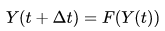——显式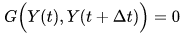——隐式

从中可看出，隐式还多了一步，解方程。

还有两者结合的显式-隐式法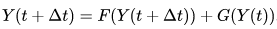举例——前向欧拉法、反向欧拉法、CN法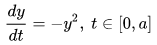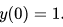前向欧拉法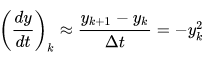即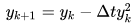式1

反向欧拉法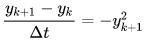即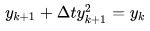可以看出有两个根，但因为初始条件为正，取正根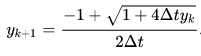式2

Crank Nicolson法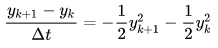即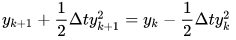举例——前向-反向欧拉法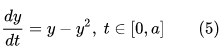前向-反向欧拉法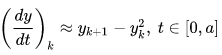即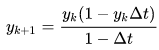CFL条件

偏微分方程收敛的条件，现在多用于差分方程收敛的条件。
时间步长Δt、空间步长Δx、速度：u、Courant number： C
显示方法Cmax=1，隐式方法允许Cmax取大点。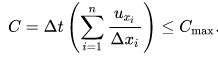满足这个条件，才会收敛。

advection的解释
advection:可以理解为平流，主要由物质浓度存在梯度引起的变化。
convection：可以理解为对流，主要是由热量不均匀引起的垂直方向的对流。

https://wenku.baidu.com/view/bb238e5a2b160b4e767fcf96.html看到篇文章推荐一下 https://blog.csdn.net/wu_nan_nan/article/details/53173302

展开全文• 在本文中，我们为时间分数扩散方程构造了一类半隐式差分方法-群显式（GE）差分方案，它是使用Saul'yev非对称方案构造的具有良好并行性的差分方案。 通过数学归纳法分析了时间分数扩散方程的GE格式的稳定性收敛性。...
• ## 显式方法与隐式方法

万次阅读 多人点赞 2013-10-18 14:48:03
显式求解是对时间进行差分，不存在迭代收敛问题，最小时间步取决于最小单元的尺寸。过多过小的时间步往往导致求解时间非常漫长，但总能给出一个计算结果。解题费用非常昂贵。因此在建模划分网格时要非常注意。...
简介
所谓显式和隐式，是指求解方法的不同，即数学上的出发点不一样。并不是说显式只能求动力学问题，隐式只能求静力学问题，只是求解策略不通。
显式求解是对时间进行差分，不存在迭代和收敛问题，最小时间步取决于最小单元的尺寸。过多和过小的时间步往往导致求解时间非常漫长，但总能给出一个计算结果。解题费用非常昂贵。因此在建模划分网格时要非常注意。
隐式求解和时间无关，采用的是牛顿迭代法（线性问题就直接求解线性代数方程组），因此存在一个迭代收敛问题，不收敛就的不到结果。
两者求解问题所耗时间的长短理论上无法比较。实际应用中一般感觉来说显式耗时多些。由于两者解题的出发点，所以一般来说显式用于求解和时间相关的动力学问题。隐式用来求解和时间无关的静力学问题。但也不是绝对的。比如，用隐式求解时，为了克服迭代不收敛，改用显式算，但是要多给点时间，这样虽然克服了不收敛的问题，但是求解的时间费用也是相当客观的。另外，隐式也可以求解动力学问题。
显式求解与隐式在数学上说主要是在求解的递推公式一个是用显式方程表示，一个是用影视方程来表示。比如a(n)=a(n-1)+b(n-1),后一次迭代可以由前一次直接求解，这就是显示方程，如果a(n)=a(n-1)+f[a(n)]，f[a(n))为a(n)的函数，此时a(n) 不能用方程显示表示，及数学上的隐函数，一般很难直接求解，多用迭代试算法间接求解。有限元在求解动力学问题中直接积分法中的中心差分积分就是显示求解，而Newmark积分法则为隐式积分。

隐式积分求解有限元问题
假设现在一个物体已经被离散成有点个单元。

显式求解与隐式在数学上说主要是在求解的递推公式一个是用显式方程表示，一个是用影视方程来表示。比如a(n)=a(n-1)+b(n-1),后一次迭代可以由前一次直接求解，这就是显示方程，如果a(n)=a(n-1)+f[a(n)]，f[a(n))为a(n)的函数，此时a(n) 不能用方程显示表示，及数学上的隐函数，一般很难直接求解，多用迭代试算法间接求解。有限元在求解动力学问题中直接积分法中的中心差分积分就是显示求解，而Newmark积分法则为隐式积分。显式求解与隐式在数学上说主要是在求解的递推公式一个是用显式方程表示，一个是用影视方程来表示。比如a(n)=a(n-1)+b(n-1),后一次迭代可以由前一次直接求解，这就是显示方程，如果a(n)=a(n-1)+f[a(n)]，f[a(n))为a(n)的函数，此时a(n)
不能用方程显示表示，及数学上的隐函数，一般很难直接求解，多用迭代试算法间接求解。
有限元在求解动力学问题中直接积分法中的中心差分积分就是显示求解，而Newmark积分法则为隐式积分。显式求解与隐式在数学上说主要是在求解的递推公式一个是用显式方程表示，一个是用影视方程来表示。比如a(n)=a(n-1)+b(n-1),后一次迭代可以由前一次直接求解，这就是显示方程，如果a(n)=a(n-1)+f[a(n)]，f[a(n))为a(n)的函数，此时a(n) 不能用方程显示表示，及数学上的隐函数，一般很难直接求解，多用迭代试算法间接求解。有限元在求解动力学问题中直接积分法中的中心差分积分就是显示求解，而Newmark积分法则为隐式积分。

首先定义几个变量：      x ： 当前物体位置；则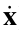表示速度，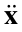表示加速度。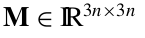为物体的质量矩阵，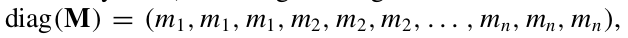对于牛顿第二定律： F=ma，用矩阵的形式表示的话: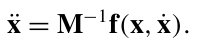其中：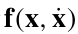表示物体在X位置下，速度为X导的情况下的受力。

首先问题的初始条件是初始位移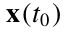和初始速度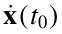。
目的是求得h时间后的位移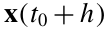和速度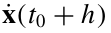。

对于式子：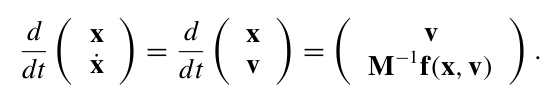若用显示欧拉求解，记，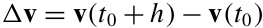，则可得到：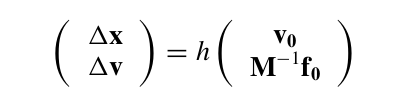这样，就利用欧拉前向方法在小步长的条件下求得当前状态的位置和速度。

对于欧拉隐式解法，上式就变成了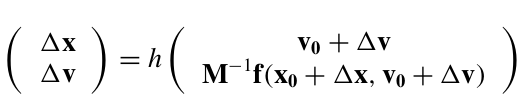前向解法和后向解法的区别就是前向的求解只依赖于前一时刻的状态量，而后向求解则不仅依赖于前一时刻的状态，还依赖于当前时刻的状态。
上式是一个非线性方程，不好直接求解，先对 f 进行泰勒级数展开：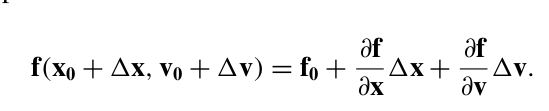代入原式：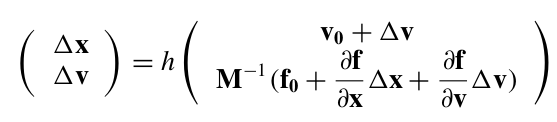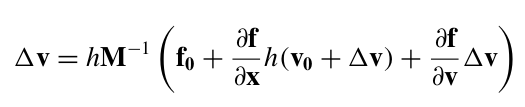用 I 表示单位矩阵，对上式进行移项，有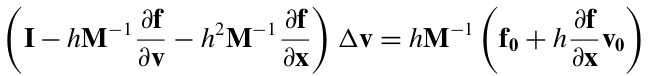通过这个式子很容易求得 detaV，然后detaX也可以求出，那么当前时刻的状态就求出来了。

参考
Large Steps in Cloth Simulation

展开全文• 显式算法与隐式算法的区别 1显式算法 最大优点是有较好的稳定性 动态显式算法采用动力学方程的一些差分格式如广泛使用的 中心差分法线性加速度法Newmark 法 wilson 法等不用直 接求解切线刚度不需要进行平衡迭代...
• 本文针对高超声速非平衡流的计算中的大时间步长，研究了一种名为ETDRK3的三阶指数时间差分方案。 二阶Harten-TVD方案用于空间离散化。 建立了雅可比矩阵对角线化方案的有效实现，并对半圆柱绕流进行了求解。 当前的...
• 通过使用隐式-显式后向差分方法时间半离散化，可以对该方程进行数值求解。 为了说明我们方法的有效性，还证明了时间半离散化方案的稳定性。 最后，我们通过一个仿真实例来说明该方法的有效性。
• ＡＢＡＱＵＳ软件Ｅｘｐｌｉｃｉｔ模块应用中心差分法对运动方程进行显式时间积分， 运动方程的解为 ¨ｕ（ｉ）＝Ｍ－１·（Ｆ（ｉ）－Ｉ（ｉ）） （１） 式中：Ｍ 为集中质量矩阵；Ｆ 为外荷载向量；Ｉ 为单元...

１显式算法
显式算法基本假定为：在一微小时间段内，模型任意点速度、加速度为常数。ＡＢＡＱＵＳ软件Ｅｘｐｌｉｃｉｔ模块应用中心差分法对运动方程进行显式时间积分，运动方程的解为¨ｕ（ｉ）＝Ｍ－１·（Ｆ（ｉ）－Ｉ（ｉ）） （１）式中：Ｍ 为集中质量矩阵；Ｆ 为外荷载向量；Ｉ 为单元内力向量。由于显式算法中不需要对刚度矩阵求逆，集中质量矩阵为对角矩阵，求逆简便，使显式算法并行计算数据传输量较小；且显式算法刚度矩阵大小与自由度数成线性关系，因此显式算法用于自由度数庞大的数值计算时具有很大优势。

2 隐式算法
隐式算法含义为：ｔ＋Δｔ时刻状态不仅与ｔ时刻状态有关，且与ｔ＋Δｔ时刻某些量有关。ＡＢＡＱＵＳ软件Ｓｔａｎｄａｒｄ 应用Ｈｉｌｂｅｒ－Ｈｕｇｈｅｓ－Ｔａｙｌｏｒ 隐式算法、Ｎｅｗｔｏｎ－Ｒａｐｈｓｏｎ迭代法进行动力方程求解［１０］。运动方程解为
Δｕ（ｉ＋１）＝Δｕ（ｉ）＋Ｋt－１ ·（Ｆ（ｉ）－Ｉ（ｉ）） （２）
式中：Ｋｔ为当前切线刚度矩阵：Δｕ为位移增量。

求解方程位移增量Δｕ（ｉ＋１）时，必须对刚度矩阵Ｋ求逆。当自由度数非常庞大时，这项计算消耗资源。对Ｋ 矩阵的求逆计算，计算机之间数据传输量非常大，随着自由度数增加，刚度矩阵Ｋ 大小成指数增长。因此，隐式算法用于自由度数庞大的数值计算时，优势不明显，甚至会降低计算效率。

根据地铁地下结构抗震研究需要，对有限元并行计算显式算法和隐式算法计算精度和效率进行比较，
主要结论如下：
（１）有限元并行计算中心差分显式算法与Ｈｉｌｂｅｒ－Ｈｕｇｈｅｓ－Ｔａｙｌｏｒ隐式算法计算精度相当，显式算法计算效率远高于隐式算法。
（２）黏弹性人工边界在显式算法和隐式算法中，都能起到很好的模拟效果。
（３）显式算法和隐式算法计算相对位移、相对速度时程基本一致，两种算法峰值应力差在９％以内。
（４）地铁地下结构抗震分析中，对于自由度很大的三维结构，并行计算显式算法计算效率较高；而对于自由度较小的二维结构，并行计算隐式算法计算效率较高。
（５）显式算法适合多处理器并行运算，对庞大自由度的地下结构三维非线性抗震分析，具有较好的并行计算效率。对于ＣＰＵ 具体使用数和计算模型自由度的关系，尚需进一步研究。

展开全文算法
• 显示和隐式区别在于，求解偏微分方程时所采用的数学策略的区别，具体而言，显示是差分隐式是牛顿迭代。求解方式的差异，必然会有不同的优缺点。 隐式 -- 优点是准确，对步长无要求（即，对网格无要求），但存在...
• 高分辨率格式 特征线与差分格式 向前向后差分格式 加权隐式格式 三层显式格式 三层隐式格式 跳点格式
• 几种典型的显示差分格式及对N—S方程的两种，段妮妮，，有限差分法是数值求解微分方程的一种重要工具，按离散方式不同可分为显式差分和隐式差分两种形式。本文主要介绍了显式差分格式的
• 非线性Huxley扩散方程的显-隐隐-显差分方法，高迪，杨晓忠，扩散方程是数学物理中一类重要的偏微分法方程, 其数值解的研究有重要的理论实际意义. 结合显式(Explicit, E)和隐式(Implicit, I)差分方法
• 此法可以认为是对中心差分法的改进,克服了中心差分法在计算阻尼矩阵为非对角阵时退化为隐式算法的缺点。中心偏心差分法的算法精度为二阶。对此算法的稳定性进行了分析。分析表明,与同类显式算法相比,本方法具有时间...
• 本文提出了一种具有并行性质的数值方法，即时间分数次扩散方程的交替分段显式-隐式（ASE-I）和隐式-显式（ASI-E）差分方法。 它基于显式方案，隐式方案，改进的Saul'yev非对称方案交替段技术的组合。 理论分析...
• 一维 Burgers 方程使用显式空间离散化（迎风中心差分）和域 (0,2) 上的周期性边界条件求解。 二维情况在 2X2 的方形域上求解，并且对扩散项使用显式和隐式方法。 沿域的边缘使用Dirichlet边界条件。matlab
• 使用 5 点有限差分模板，使用隐式矩阵求逆技术和显式迭代解法在 2d 中求解拉普拉斯方程。 使用的边界条件包括 Dirichlet 和 Neumann 类型条件。matlab
• 第10节 显示有限差分法计算期权价格 10.1 简介 10.2 计算美式看跌期权价格算法 ...显式和隐式差分法的区别主要在于替代计算微分方程的差分方程中对微分项的离散化近似表示不同（主要是∂f∂t\frac{\partial fpython 算法
• 采用斜向差分算子［1］，建立斜向隐式差分格式，再结合边界条件，对扩散方程进行求解。此算法虽然是隐格式，但可以利用边界条件显式计算，既保持了隐格式的稳定性精度，也减少了计算复杂性。通过具体的数值算例...
• OpenFiniteDifference 一维有限差分法（对流扩散方程）。 此处包括显式隐式和Crank-Nicolson方法。 在这里，PETSc用于求解线性方程。C++
• 对于二维对流扩散方程,利用一阶二阶导数的四阶Padé型紧致差分逼近式,结合原方程,得到了求解该方程的一种四阶精度的隐式紧致差分格式。该格式在每个空间方向上只涉及到3个点处的未知量及导数值,对导数利用四阶显式...
• 该方法由于利用了随机差分矩阵，因此有很好的安全特性，并能很好地实现显式或者隐式水印信息的加载。实验结果显示，该方法能在不影响原始图像的观感条件下实现水印的有效添加，并且所添加的水印图像能有效地防止噪声...数字水印 信噪比
• 使用各种有限差分方案（Lax-Friedrichs，Lax-Wendroff，MacCormack具有四阶显式人工黏度项的隐式Beam-Warming）以保守形式模拟非线性对流方程。 所使用的初始条件是Heaviside阶跃函数，并且预计该波将向右传播（平...matlab
• black_scholes_naive_implicit.m-隐式有限差分方法在基本方程组上的应用。 black_scholes_cov_explicit.m-该文件涉及使用变量更改以将PDE强制转换为热方程式。 然后，我们对结果方程应用显式有限差分方法。 ...MATLAB
• 1. 动静板间的流体流动... 二维线性化伯格方程二维椭圆拉普拉斯方程FTCS对流显式为一阶上风，而对扩散为二阶中心。 雅可比角带 SOR 的高斯-赛德尔5.盖子驱动的腔流，流函数-涡度公式墙壁入口/出口。 流函数 BC。matlab
• 该程序采用有限差分方法（隐式和显式）仿真了一维和二维域扩散方程。该程序采用有限差分方法（隐式和显式）仿真了一维和二维域扩散方程。该程序采用有限差分方法（隐式和显式）仿真了一维和二维域扩散方程。
• 该程序采用有限差分方法（隐式和显式）仿真了一维和二维域扩散方程。该程序采用有限差分方法（隐式和显式）仿真了一维和二维域扩散方程。该程序采用有限差分方法（隐式和显式）仿真了一维和二维域扩散方程。
• 隐式格式的MATLAB代码 A 2D heat transfer case study for the Food Processing Engineering course ...代码中提供了显式和隐式两种 FDM 差分格式。 How to build fire matlab run install.m run main.m